# Introduction to clustering: the K-Means algorithm (with Java code)

In this blog post, I will introduce the popular data mining task of clustering (also called cluster analysis).  I will explain what is the goal of clustering, and then introduce the popular K-Means algorithm with an example. Moreover, I will briefly explain how an open-source Java implementation of K-Means, offered in the SPMF data mining library can be used.

What is clustering?

Clustering is one of the most fundamental data mining tasks.

Consider that you have a database, containing several records (which we will call instances). For example, consider the following database describing several bank customers.

```AGE     NATIONALITY    INCOME   GENDER   ACCOUNT_BALANCE  ...
42      Brazil          4000     F         500
...```

In this example, each instance (customer) is described according to some attributes such as agenationalityincome, gender, and  account_balance.

The goal of clustering is to automatically find groups of instances (e.g. customers) that are similar in a database. For example, for the database of bank customers, clustering the customers, consists of automatically grouping the customers that have a similar profile. For example, a clustering algorithm could perhaps find that many female customers who are rich have a similar profile or that many young Canadians with a low income have a similar profile (this is just an example).

Thus, the goal of clustering is to find groups of instances having similar characteristics in a database. These groups of instances found by clustering are called clusters. Thus, the goal of clustering is to find clusters of similar instances.

Let’s have a look at a second example before giving a more formal definition of what is clustering.  This time, we will consider a database of  2D points.  Consider the following database containing 31 two dimensional points.

 Instance 1 (1,1) Instance 17 (16, 16) Instance 2 (0,1) Instance 18 (11.5, 8) Instance 3 (1,0) Instance 19 (13, 10) Instance 4 (11,12) Instance 20 (12, 13) Instance 5 (11, 13) Instance 21 (14, 12.5) Instance 6 (13, 13) Instance 22 (14.5, 12.5) Instance 7 (12, 8.5) Instance 23 (14.5, 11.5) Instance 8 (13, 8) Instance 24 (15, 10.5) Instance 9 (13, 9) Instance 25 (15, 9.5) Instance 10 (13, 7) Instance 26 (12, 9.5) Instance 11 (11, 7) Instance 27 (10.5, 11) Instance 12 (8, 2) Instance 28 (10, 10.5) Instance 13 (9, 2) Instance 29 (9, 3) Instance 14 (10, 1) Instance 30 (9, 4) Instance 15 (7, 13) Instance 31 (9,5) Instance 16 (5, 9)

Each instance (point) in this database is described by two attributes:  the X and Y coordinates.  This database can be represented visually usually a XY chart: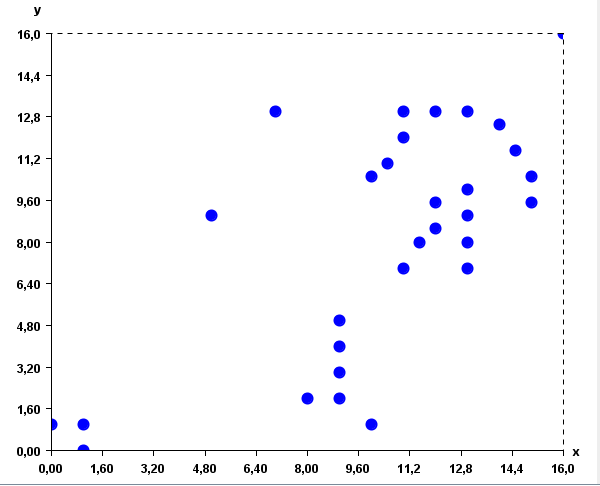It can be observed in these figures that some points are quite close, while some other points are quite far away. In this context, the goal of clustering is to find groups of points that are similar (close to each other).  By applying a clustering algorithm such as K-Means, three clusters could be found (represented by the blue, red and green colors):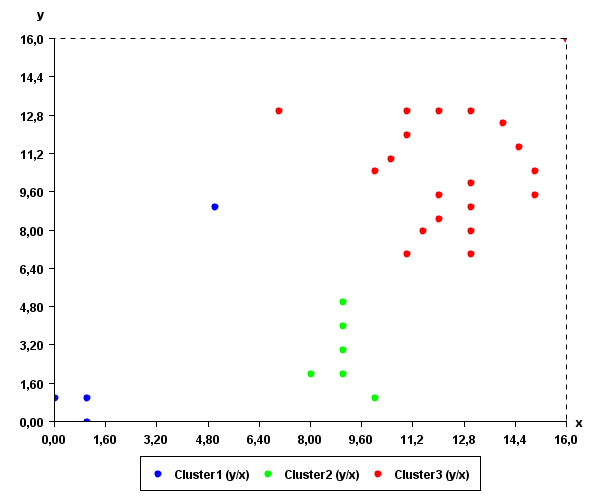Intuitively, these clusters somewhat make sense as they are groups of points that are close to each other.

Clustering has many applications. It can be used for example to cluster similar documents in categories (for example, automatically classifying news on a website into categories).

Clustering algorithms

To perform clustering, it is necessary to apply a clustering algorithm. There exists hundreds of clustering algorithms to find hidden clusters in data.  Different algorithms have different advantages and disadvantages and can be more or less suitable to different types of data.  Some of the most popular algorithms are for example K-Means and DBScan.

In the following paragraphs, I will briefly explain how the K-Means algorithms works.

The K-Means algorithm

The K-Means algorithm was proposed in 1967 by MacQueen.  This algorithm has two main parameters:  (1) a database,  (2)  a positive integer K representing the number of clusters to be extracted from the database.

The K-Means algorithm consists of the following steps:

(1) The algorithm reads the database in memory. The database contains several instances.

(2) The algorithm initialize K empty clusters. Each cluster has a prototype, which is a randomly generated instance.

(3)  Each instance in the database is assigned to the cluster  having the closest prototype.

(4) Then, the prototype of each cluster is recomputed as the average of all the instances in that cluster.

(5) Then, Step3 and Step 4 are repeated several times, until the clusters become stable.

Now let me illustrate these steps with an example so that it becomes clear. By applying K-Means on the database of 2D points, (1) K-Means will first load the database of 31 points in memory. Then, assume that the user has set K = 3 to generate 3 clusters. (2) K-Means will initially create three empty clusters which will have some random points as prototypes.  Let say that these three points (prototypes) are (2,2),  (16, 4) and (11, 0) .  We can represent this visually as follows (where the prototypes are represented by the X symbol):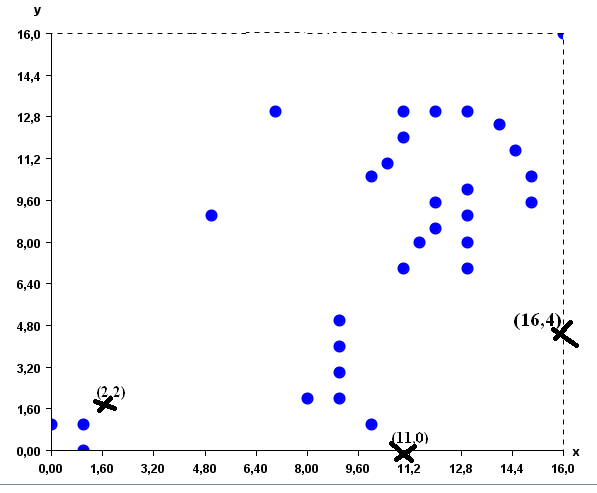Then (3) K-means will assign each of the 31 points to the cluster having the closest prototype. The result will be as follows:Then (4) the prototype of each cluster is recomputed as the average of the points that it contains. The new prototypes are approximately (1.75, 2.75), (9.1, 5.2) and (12.9, 10.7) for the three clusters of points.Then (3), each point is assigned to the cluster having the closest prototype. The result is the clusters shown in the picture below. As you can see, some points have moved from one cluster to another.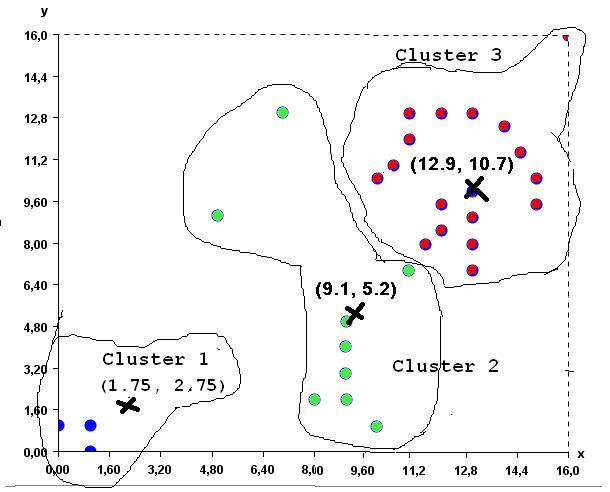Then, step (4) is applied again to recompute the prototype of each cluster as the average of its points. The new prototypes are (0.7, 0.7), (12.4,10.8) and  (8.7,4.1).

Then step (3) is applied again. K-Means assigns each point to the cluster having the closest prototype. Since the cluster did not change after this step, the K-Means algorithms stop and the final result is the following three clusters, here displayed with colors:An open-source Java implementation of K-Means

If you want to try the K-Means algorithm with the above example by yourself,  a Java implementation of K-Means is provided in the SPMF library.

To run the example, you will need to have Java 1.8 or higher installed on your computer.

(1) The first step is to download the database of points, which can be obtained here:

http://www.philippe-fournier-viger.com/spmf/inputDBScan2.txt

(2) Then, you will need to download the release version of SPMF, which is a file called spmf.jar, and can be obtained from the download page of SPMF.

(3) Then, double-click on the file spmf.jar to launch SPMF. If it does not work, it means that there is a problem with the Java installation on your computer.  If it works correctly, the user interface of SPMF should open: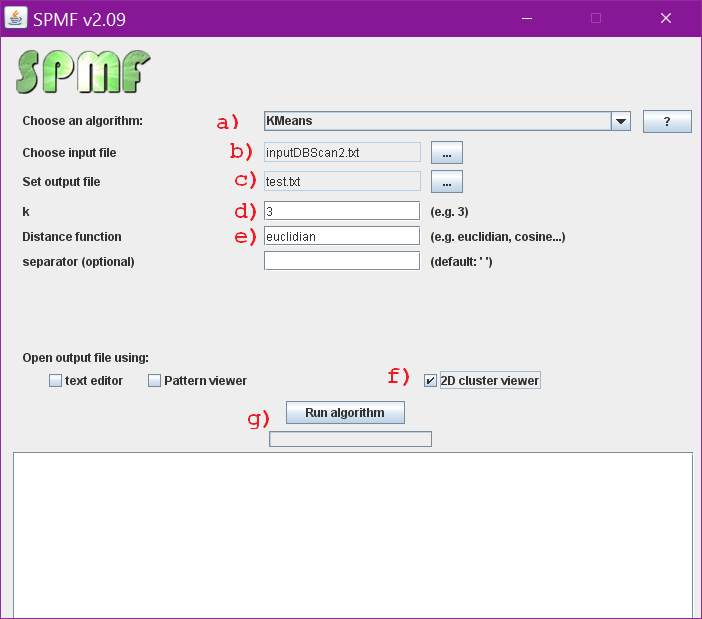• (A)  choose the KMeans algorithm in the combo box.
• (B)  choose the input file  as  “inputDBScan2.txt”
• (C) set the output file as “text.txt”. The output of the algorithm will be written to that file.
• (D) Set the parameter K to 3
• (E) To be able to calculate how similar the instances are close to each other, we need to specify a distance function. For this example, we should use the Euclidian distance, as it is the typical distance function for comparing 2D points.
• (F) Select the “cluster viewer” to see the results as a chart.
• (G) Click the “Run algorithm” button” to launch the K-Means algorithm.

The result will be displayed in a window, such as this: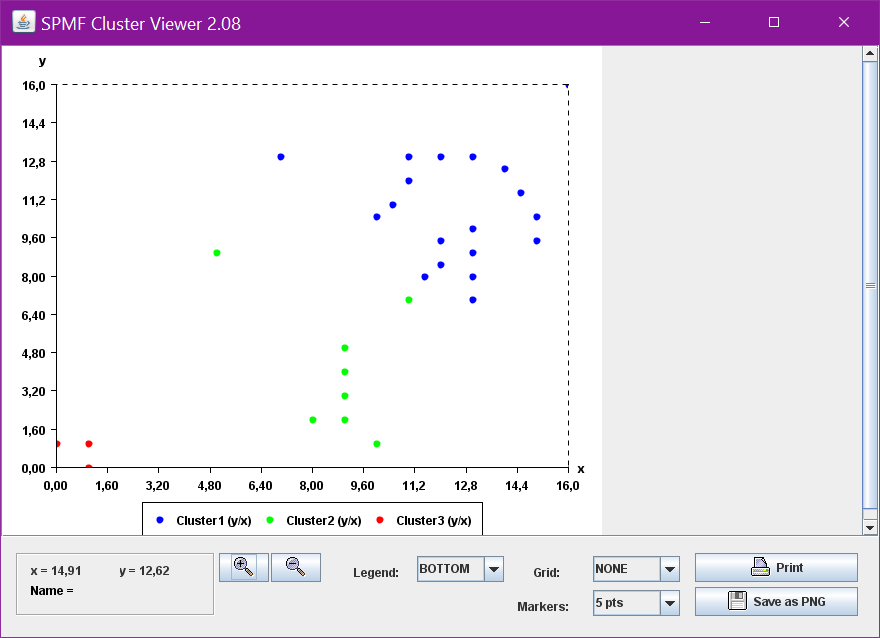Note that it is quite possible that the result that you will obtain is not exactly the same. The reason is that K-Means is a randomized algorithm. In other words, K-Means utilize random numbers. Thus, if K-Means is run several times, it may not always generate the same result.

Why is the K-Means algorithm popular?

Having presented the K-Means algorithm, let’s now briefly discuss its characteristics in more details. Why is K-Means popular?  The main reason is that it is a very simple algorithm. It is easy to implement and also easy to understand.

Some drawback of the K-Means algorithms is that the final result depends on how the initial prototypes are selected randomly. If we are lucky, we may find some nice clusters as in the above example, that somewhat make sense. However, if the initial points are not chosen in an appropriate way, the result may not be meaningful. There exists various solutions to this problem such as running K-Means several times, and then to choose the best set of clusters.

Another limitation of K-Means is that the user must explicitly specify the number of clusters to be found (the K parameter). But finding the best value for the K parameter may require to try several values.

To address these limitations various extensions of K-Means have been proposed. Besides, many other clustering algorithms have been proposed.

Conclusion

This blog post has given an overview of the task of clustering, a fundamental data mining task. The goal was to give a simple introduction. The post has explained how the K-Means algorithm works and has shown how to use the SPMF implementation of K-Means. In a future blog post, I will explain the DBScan clustering algorithm, which is also offered in SPMF.

For the interested reader, a good introduction to clustering can be found in the book “Introduction to data mining” by Tan, Steinbach and Kumar. The first chapter about clustering is freely available here.

Philippe Fournier-Viger is a full professor and also the founder of the open-source data mining software SPMF, offering more than 110 data mining algorithms. If you like this blog, you can tweet about it and/or subscribe to my twitter account @philfv to get notified about new posts.

(Visited 15,653 times, 1 visits today)

### Related posts:

#### Introduction to clustering: the K-Means algorithm (with Java code) — 31 Comments

1.nigam on said:

• As explained in the blog post, all the code is available on the SPMF website: http://www.philippe-fournier-viger.com/spmf/

There you can go to the download page and download the source code of SPMF to find the code of KMeans and you can modify it if you like.

Or you can download the JAR file and run SPMF as a software.

The source code is quite simple to understand. If you are familiar with Java it should not be easy to understand my code.

But don’t forget to read the installation instructions on the download page. It explains everything. And there is also some documentation on the webpage that provides an example of how to run each algorithm.

2.Khalid Aliyu Muhammad on said:

Great work sir, just started following as my interest toward learning more on data mining grows. thanks

3.Danny Coutts on said:

Hi Philippe

I am a geologist who is new to clustering. I appreciate of your explanation of K-means. Its definitely one of the best I’ve read.

I have a dataset of x-y data. x is an easily measurable property, whereas the data that composes our y is a dataset of very time consuming and expensive measurements. K-means and other clustering algorithms cluster the x-y data in to clusters that are defined along both the x and y of the data. I would like to cluster our x-y data into the optimal number of bins along the x axis based the x-y data. This would allow me to derive a range of measurements for modelling the values of y from the descritized classes of x.

Do you have any suggestions on how to possibly do this?

• Dear Danny,

Thanks for reading the blog. It is interesting that you are considering applying clustering to geological data.

For your question, I assume that X and Y are numerical values. You could first test to see if there is really a strong correlation between the values of X and the values of Y by calculating something like the Pearson Correlation (this can be done using Excel) for example, and would give you some idea if X and Y are correlated or not.

You could also consider training some models that can predict the value of Y based on the value of X. Assuming that there is some correlation between X and Y, it may be possible to quite accurately predict the value of Y based on X. For example, this could be done using neural networks, or something more simple like logistic/linear regression. In that case, you can train a model with some values of X,Y that you already know. Then, using your trained model, you can predict the value of Y for some new values of X.

Now, if you want to apply clustering, a possibility is to modify K-Means to cluster all your X,Y values using only the values of X, or using only the values of Y. Maybe that it could be used to make some bins. But not sure if it would work well. If there is some strong correlation between X and Y maybe you could get something good. But it may not give you something “optimal”

4.Pradhuman on said:

Such a nice introduction of KMeans.

Thank you for writing.

5.Sourav Das on said:

Respected Sir
I Have Download All Zip File But I Can’t Run Graphical Interface
So Can u Help Me How To Run

• Hello, If You Want To Run The User Interface You Should Download The Jar File. The Jar File and Instructions About How To Run the User Interface Are On The Download Page of SPMF. Everything is Explained There.

6.Asha on said:

Well written article. thanks

7.Shalini on said:

hi sir,thankyou for providing us such a understandable explanation of k-means.
Sir can i get the source code for k-m0des algorithm in java since its extention of k-means??

• Hi,

If It is simple to implement, maybe I can implement it. Can you send me the paper at philfv8 AT YAHOO DOT COM ? Then, I will have a look. But these days I am very busy so I cannot guarantee that I will do it. Thanks

8.Surya on said:

Dear Philippe

I really need this source code for my essay, so thanks, i’m grateful to find your code.
but i have question, I have 4 values to calculate, not a “X,Y” values, how to implement your code for 4 values, thanks..

sincerely
Surya.

• Hi,

Glad it is useful. My code works for any number of values. I use two values for the example. But you can use 4, 5, or as many values as you need.
It is the same input file format. You just add more values on each line.

Best,
Philippe

9.Syed Mujahed on said:

Dear sir,
Very nice explanation of K-Means algo. Thank you very much for explaining in an easy way with an example. Sir, I have project in text mining for concept labeling (semantic labeling to the short text based on context). Could you please help me in this regard. I need java code to implement this logic.
Thanking you

• Hi,
Glad you like the blog post about k-means. I tried to write an introduction that is easy to understand.

I do not have code for that and do not have time to implement this because I am very busy. All the code that I have is in the SPMF library. If it is not there, then I do not have it.

Best regards,

10.Jiyeon An on said:

Dear Phiippe
Thank you for offering such a nice posting about k-means.
We’re using this SPMF system for our group work.
But it doesn’t work when we put over 8 into k.
Can you give us some suggestions? or way to operate our data?

• Hi,

Thanks for using SPMF and glad that the post is useful. Could you tell me more precisely what you mean by “not working”? What is the result that you expect? And what is the problem that you are getting If the problem is that some clusters are empty, then it is actually normal in some way. Actually K-Means is a randomized algorithm that randomly select some cluster centers to initialize the clusters. Then depending on these centers, it is possible that some clusters are empty.
Best,
Philippe

11.ashwini sadhu on said:

where is code explained?

12.Praveen on said:

Dear Sir,
This is Praveen Research scholar in Kakatiya university Warangal,India
Sir My PhD Title is A Heurstic Tree Structured Approach to Improve Efficiency of Clustering Data.
Could you send Clustering All Algorithms Programs in Java.

13.NthaLebaka on said:

how can i use kmeans in php for disease prediction system?

• You could implement K-means in PHP and then find data about disease. Then you could cluster the data to find people having similar profiles. Then to perform a prediction for a new person, you could compare that person with your clusters, and then make the prediction.

14.xyz on said:

how can i use clustering algorithm to cluster a set of real life data based on certain parameters other than distance?

15.mamatha on said:

16.Maciej Muras on said:

Hello,
thank you for good explaination. What would be distance function for tasks related to big data for example for twitter data?

• Hello, glad you like it. Twitter data is text. Some basic measure for text is the edit distance. However, if you want to compare text at a semantic level rather than in terms of characters, you could consider using something based on LSA (Latent Semantic Analysis for example. In LSA, words will be represented in a vector space based on their meaning, and then you can calculate the distance in terms of semantics. But I did read about that for a while, so maybe there also exists some newer techniques, but you could check that as a starting point. Regards

•Maciej Muras on said:

Hello Phillipe,
i created program for getting tweets suitable to written query, but here are problems too. Tweet data is very dirty and it’s next problem to clean it. I’ve been looking for java library for text vectorization and i found Dl4j using deep learning methods for finding semantic similarity between ‘paragraphs’. Reffering again to k-means

‘(4) Then, the prototype of each cluster is recomputed as the average of all the instances in that cluster.’)’

i’m not sure how to recompute prototype having vector instances which represent text paragraph. Maybe you have idea how to resolve this.

Regards,
Maciej

17.chalapathi on said:

sir cam I run my own algorithm

• Which algorithm do you want to run?